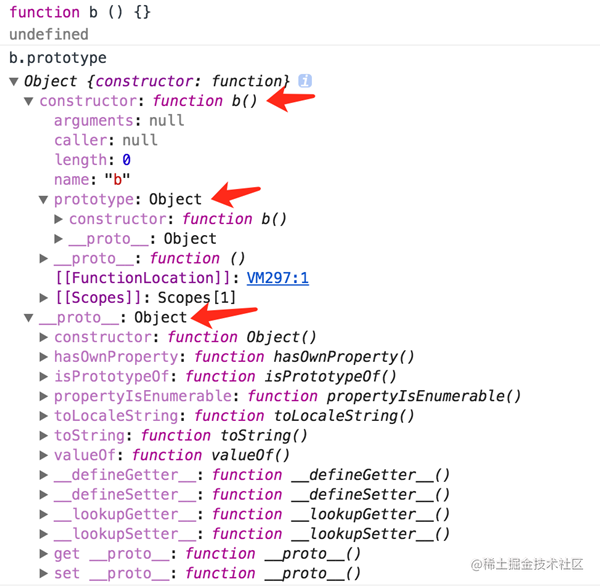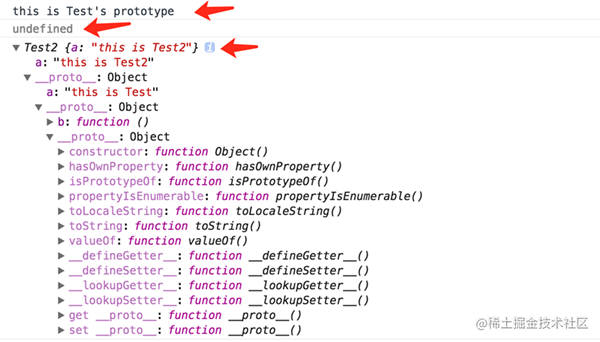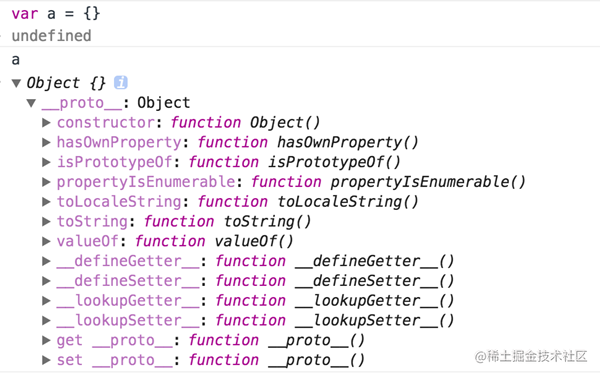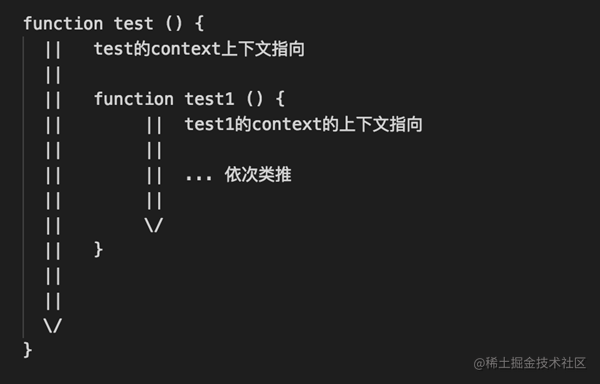# 一、this

this是什么？其实它本身就是一种指向。this指向可以分为以下几种情况

• 普通调用，this指向为调用者
• call/apply调用，this指向为当前thisArg参数
• 箭头函数，this指向为当前函数的this指向

## 1、普通调用

### 1.1、对象方法的调用

``````var obj = {
a: 'this is obj',
test: function () {
console.log(this.a);
}
}
obj.test();
// this is obj复制代码``````

### 1.2、“单纯”函数调用

``````var a = 'this is window'
function test () {
console.log(this.a);
}
test();
// this is window复制代码``````

``````window.a = 'this is window'
window.test = function test () {
console.log(this.a);
// 此时是window为调用者，即this会指向window
}
window.test();复制代码``````

### 1.3、构造函数调用

``````function test () {
this.a = 'this is test';
console.log(this.a);
console.log(this);
}
test();
// this is test
// Window {}复制代码``````

``````function Test () {
this.a = 'this is test';
console.log(this.a);
console.log(this);
}
var test = new Test();
// this is test
// Test {a: 'this is test'}复制代码``````

OK，好像的确没有问题了，此时的this的确指向了该构造函数的实例对象。具体这里的一些解释后面我会在原型链继承里面详细讲解。

## 2、call/apply调用

### 2.1、call调用

call方法形式，fun.call(thisArg[, arg1[, arg2[, ...]]])

• thisArg，当前this指向
• arg1[, arg2[, ...]]，指定的参数列表

``````function Test () {
this.a = 'this is test';
console.log(this.a);
console.log(this);
}
function Test2 () {
Test.call(this);
}
var test = new Test2();
// this is test
// Test2 {a: 'this is test'}复制代码``````

### 2.2、apply调用

• thisArg，当前this指向
• 一个数组或者类数组对象，其中的数组元素将作为单独的参数传给fun函数

## 3、箭头函数

``````function test () {
(() => {
console.log(this);
})()
}
test.call({a: 'this is thisArg'})
// Object {a: 'this is thisArg'}复制代码``````

``````function test () {
setTimeout(() => {
console.log(this);
}, 0)
}
test.call({a: 'this is obj'})
// Object {a: 'this is obj'}复制代码``````

``````function test () {
setTimeout(function () {
console.log(this);
}, 0)
}
test.call({a: 'this is obj'})
// Window {...}复制代码``````

# 二、原型/原型链

• 原型：即每个function函数都有的一个prototype属性。
• 原型链：每个对象和原型都有原型，对象的原型指向原型对象，而父的原型又指向父的父，这种原型层层连接起来的就构成了原型链。``````function Test () {
this.a = 'this is Test';
}
Test.prototype = {
b: function () {
console.log("this is Test 's prototype");
}
}
function Test2 () {
this.a = 'this is Test2 '
}
Test2.prototype = new Test();
var test = new Test2();
test.b();
console.log(test.prototype);
console.log(test);复制代码``````# 三、继承

## 1、构造函数继承

``````function Test () {
this.a = 'this is test ';
console.log(this.a);
console.log(this);
}
function Test2 () {
Test.apply(this)
// or Test.apply(this)
}
var test = new Test2();
// this is test
// Test2 {a: 'this is test '}复制代码``````

## 2、原型链继承

``Child.prototype = new Parent();复制代码````````var obj= {}; // 初始化一个对象obj
obj.__proto__ = Parent.prototype; // 将obj的__proto__原型指针指向父类Parent的prototype属性
Parent.call(obj); // 初始化Parent构造函数复制代码``````

``````function Parent () {
this.a = 'this is Parent '
}
Parent.prototype = {
b: function () {
console.log(this.a);
}
}
function Child () {
this.a = 'this is Child '
}
Child.prototype = {
b: function () {
console.log('monkey patch ');
Parent.prototype.b.call(this);
}
}
var test = new Child()
test.b()
// monkey patch
// this is Child复制代码``````

# 四、闭包

JavaScript语言特性，每一个function内都有一个属于自己的执行上下文，即特定的context指向。``````var a = 'this is window '
function test () {
var b = 'this is test '
function test2 () {
var c = 'this is test2 ';
console.log(a);
console.log(b);
console.log(c);
}
test2();
}
test();
// this is window
// this is test
// this is test2复制代码``````

``````function test () {
var b = 'this is test
';
}
console.log(b); // Uncaught ReferenceError: b is not defined复制代码``````

``````function test () {
for (var i = 0; i < 4; i++) {
setTimeout(function () {
console.log(i);
}, 0)
}
}
test();复制代码``````

``````function test () {
for (var i = 0; i < 4; i++) {
(function (e) {
setTimeout(function () {
console.log(e);
}, 0)
})(i)
}
}
test();
// 0 -> 1 -> 2 -> 3复制代码``````

``````function test () {
for(var i = 0; i < 4; i++) {
setTimeout((function(e) {
return function() {
console.log(e);
}
})(i), 0)
}
}
test();复制代码``````